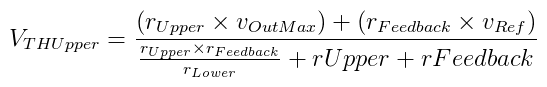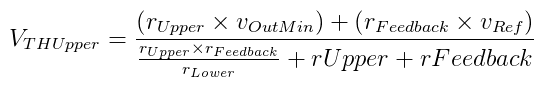# The Lab Book Pages

An online collection of electronics information

Dr. Andrew Greensted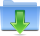Comparator.jar

# Comparator Hysteresis Network

The Comparator Network Search Tool is used to find a set of resistor values in an inverting comparator circuit that provides the required switching threshold voltage and hysteresis. The reverse can also be performed, finding threshold and hysteresis voltages based on known resistor values. The application can be started using Java Web Start by clicking the Launch button below. Alternatively, a executable Jar file can be downloaded using the link above.

## Inverting Comparator Circuit

The inverting comparator circuit analysed by the search tool is shown in the left-hand figure below. The feedback resistor provides hysteresis to the switching threshold voltages. The right-hand image below shows how the output voltage changes when the input crosses the switching thresholds.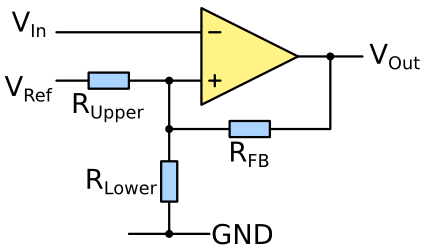Inverting comparator circuit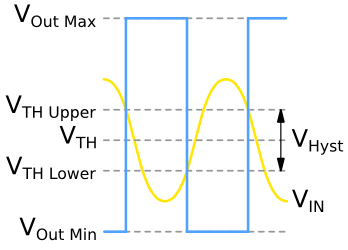Input and output voltages of inverting comparator circuit

The image below shows the importance of using a comparator circuit with some input hysteresis. The example is cleaning up a noisy waveform into a binary output signal. The input voltage waveform is shown in the lower blue waveform. The output of a simple comparator without any threshold hysteresis is shown in the top red waveform. The noise means the input crosses the centre threshold many times, so the output switches accordingly.

The middle green waveform shows the result of using threshold hysteresis. When a switching threshold is crossed, the output changes and the threshold changes as well. This means is it less likely that noise will cause the output to switch again.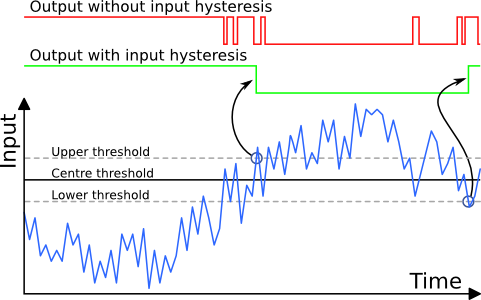Input hysteresis can help clean up noisy data

The two equations below can be used to calculate the switching thresholds based on the comparator circuit resistor values.